# Samacheer Kalvi 6th Maths Solutions Term 1 Chapter 3 Ratio and Proportion Additional Questions## Tamilnadu Samacheer Kalvi 6th Maths Solutions Term 1 Chapter 3 Ratio and Proportion Additional Questions

Question 1.
3 : 5 :: ____
Solution:
6 : 10

Question 2.
Moni walks 6 km in an hour while Vimala walks 4 km in an hour. What is the ratio of the distance covered by Moni to the distance covered by Vimala?
Solution:
Distance covered by Moni in 1 hour = 6 km
Distance covered by Vimala in 1 hour = 4 km.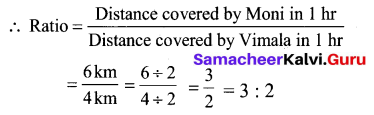The required ratio = 3 : 2

Question 3.
In a school there were 75 holidays in one year. What is the ratio of the number of holidays to the number of days in one year.
Solution:
Given the number of holidays = 75.
We know that the number of days in one year = 365.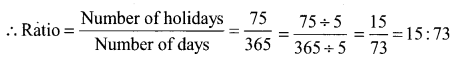Hence the required ratio = 15 : 73

Question 4.
There are 20 girls and 25 boys in a class.
(a) What is the ratio of number of girls to the number of boys?
(b) What is the ratio of number of girls to the total number of students in the class?
Solution:
Given number of girls = 20
Number of boys = 25
Total number of students = 20 + 25 = 45.
Ratio of Number of girls to the number of boys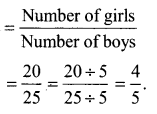girls : boys = 4 : 5
Ratio of number of girls to the total number of students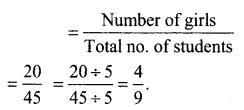Ratio of no. of gets to total students = 4 : 9Question 5.
Simplify the ratio 20 : 5
Solution:
$$\frac{20}{5}=\frac{4}{1}$$ = 4 : 1

Question 6.
Mother wants to divide ₹ 36 between her daughters Sumi and Divya in the ratio of their ages. If age of Sumi is 15 years and age of Divya is 12 years. Find how much Sumi and Divya gets?
Solution:
Given Sumi’s age = 15 years
Divya’s age = 12 years
Ratio of their ages
$$\frac{\text { Sumi’s age }}{\text { Divya’s age }}=\frac{15 \text { yrs }}{12 \text { yrs }}=\frac{5}{4}=5: 4$$
Now mother wants to divide ₹ 36 between the daughters in the ratio of their ages.
Sum of the parts of ratios = 5 + 4 = 9.
Sumi gets 5 parts and Divya gets 4 parts out of 9 equal parts.
Sumi’s share $$=\frac{5}{9} \times 36$$ = ₹ 20
Divya’s share $$=\frac{4}{9} \times 36$$ = ₹ 16

Question 7.
The ratio of breadth and length of a hall is 2 : 5. Complete the following table that shows some possible breadths and lengths of the hall.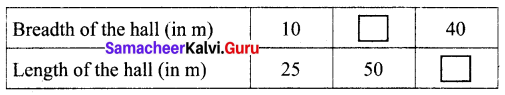Solution:
Given the ratio of breadth and length of the hall = 2 : 5.
Also given breadth of the hall = 10
length of the hall = 25.
Ratio of breadth : length = $$\frac{10}{25}=\frac{2}{5}=2: 5$$
To find First missing number:
Take the first ratio = $$\frac{2}{5}=\frac{2 \times 10}{5 \times 10}=\frac{20}{50}$$
First missing number = 20.
Also, $$\frac{2}{5}=\frac{2 \times 20}{5 \times 20}=\frac{40}{100}$$
Second missing number = 100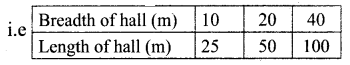Question 8.
Kumaran has ₹ 600 and wants to divide it between Vimala and Yazhini in the ratio 2 : 3, who will get more and how much?
Solution:
Divide the whole money into 2 + 3 = 5 equal parts then, Vimala gets 2 parts out of 5 parts and Yazhini gets 3 parts out of 5 parts.
Amount Vimala gets = $$600 \times \frac{2}{5}$$ = ₹ 240
Amount Yazhini gets = $$600 \times \frac{3}{5}$$ = ₹ 360
Vimala received ₹ 240 and Yazhini gets ₹ 360, which is ₹ 120 more than that of Vimala.

Question 9.
Find the value of x if 16 : 24 :: x : 30.
Solution:
Given the two ratios are in proportion.
Product of extremes = 16 × 30 = 480
Product of means = 24 × x
We know that product of extremes = product of mean 480
480 = 24 × x
⇒ x = 20Question 10.
The cost of 12 pens is ₹ 96, then find the cost of 8 such pens.
Solution:
Since the ratio of number of pens to its cost are in proportion.
We say that 12 : 96 :: 8 : cost
Product of extremes = 12 × cost
Product of means = 96 × 8 = 768
Product of extremes = product of means
12 × Cost = 96 × 8
Cost = $$\frac{96 \times 8}{12}=64$$
Cost of 8 pens ₹ 64

Question 11.
If the ratio between 72 and y is same as the ratio between 64 and x then what is the ratio between x and y?
Solution:
Given ratio between 72 and y = ratio between 64 and x
$$\frac{72}{y}=\frac{64}{x}$$
Taking cross product for one term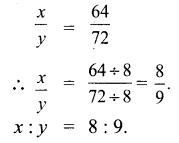Question 12.
Fill in the boxes:
(i) ___ : 24 :: 80 : 64
(ii) 5 : 6 :: 125 : ___
Solution: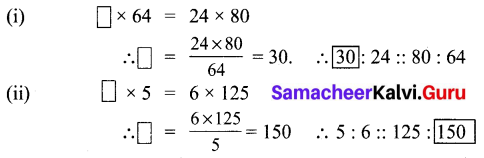Question 13.
Aadisaran made 50 runs in 10 overs and Mohan made 42 runs in 7 overs. Whose run rate is better?
Solution:
Run Rate = Ratio of runs to over
Run rate of Aadisaran = $$\frac{50}{10}$$ = 5
Run rate of Mohan = $$\frac{42}{7}$$ = 6
Mohan’s run rate is better.

Question 14.
If the cost of 7 m of cloth is ₹ 294 find the cost of 5 m of cloth.
Solution:
Given cost of 7 m of cloth = ₹ 294.
Cost of 1 m of cloth = $$\frac{294}{7}$$ = ₹ 42
Cost of 5 m of cloth = ₹ 42 × 5 = ₹ 210.
Cost of 5 m of cloth = ₹ 210.

Question 15.
Divino earns ₹ 1500 in 10 days. How much will she earn in 30 days?
Solution:
Divino’s earning for 10 days = ₹ 1500
His earning in 1 day = $$\frac{1500}{10}$$ = ₹ 150
Divino’s earning in 30 days = 150 × 30 = ₹ 4,500
Divino earns ₹ 4,500 in 30 days.

Question 16.
The temperature dropped 15 degree Celsius in the last 30 days. If the rate of temperature drop remains the same how many degrees will the temperature drop in the next 10 days?
Solution:
Temperature drop in 30 days = 15 degrees.
Temperature drop in 1 days = $$\frac{15}{30}=\frac{15 \div 15}{30 \div 15}=\frac{1}{2}$$
Temperature drop in next 10 days = $$\frac{1}{2} \times 10$$ = 5 degrees
Hence temperature dropped 5 degrees in the next 10 days.Question 17.
Shobana pays ₹ 7500 as rent for 3 months. How much does he has to pay for the whole year if the rent per month remains the same?
Solution:
Rent paid for 3 months = ₹ 7500
Rent paid in 1 month = $$\frac{7500}{3}$$ = ₹ 2500
Rent paid for the whole year = 2500 × 12 = ₹ 30,000
Shobana has to pay ₹ 30, 000 for the whole year.

Question 18.
By proportionality law, check whether 3 : 2 and 30 : 20 are in proportion.
Solution:
Here the extremes are 3 and 20 and the means are 2 and 30.
Product of extremes, ad = 3 × 20 = 60.
Product of means, bc = 2 × 30 = 60.
Thus by proportionality law, we find ad = bc and hence 3 : 2 and 30 : 20 are in proportion.